# How do I construct a straight line through data points? Best-fit lines

## Best-fit lines can also be called:

Linear regression
Trend lines
Questions that ask you to draw a best fit line or trend in the data usually do not want you to "connect the dots". Instead, the question is asking you to think about how the two sets of data behave in relation to one another. In general, we fit lines to data when we want to use them for predictive purposes or to determine the general trend of the data.

Most scientists use a computer program to plot a best-fit line for a set of data but constructing one for yourself is a good way to learn how it's done. Because a computer isn't doing it, you may find that your "best-fit" line is slightly different from your lab partners. In most cases, that is okay, as long as you've mimicked the trend of the data.

## Why (and when) should I use a best fit line?

In introductory geoscience, most exercises that ask you to construct a best-fit line have to do with wanting to be able recognize relationships among variables on Earth or to predict the behavior of a system (in this case the Earth system). We want to know if there is a relationship between the amount of nitrogen in the water and the intensity of an algal bloom, or we wish to know the relationship of one chemical component of a rock to another. For predictive purposes, we might prefer to know how often an earthquake is likely to occur on a particular fault or the possibility of a very large flood on a given river. All of these applications use best-fit lines on scatter plots (x-y graphs with just data points, no lines).

If you find yourself faced with a question that asks you to draw a trend line, linear regression or best-fit line, you are most certainly being asked to draw a line through data points on a scatter plot. You may also be asked to approximate the trend, or sketch in a line that mimics the data. This page is designed to help you complete any of these types of questions. Work through it and the sample problems if you are unsure of how to complete questions about trends and best-fit lines.

## How do I construct a best-fit line?

A best-fit line is meant to mimic the trend of the data. In many cases, the line may not pass through very many of the plotted points. Instead, the idea is to get a line that has equal numbers of points on either side. Most people start by eye-balling the data.
1. Take a look at the data and as yourself these questions
2. Now that you have an idea of the general trend of the data, there are two possible ways to construct a best-fit line by eye. You may use either of them; both are correct and relatively easy ways to get a pretty accurate representation of a best-fit line. Pick the one that makes the most sense to you. The first method involves enclosing the data in an area:
1. Begin by plotting all your data. For this example, we will use some geochemical data from Lassen Peak, a volcano in Northern California that last erupted in 1915 (the data was collected by an undergraduate research student at University of Wisconsin Oshkosh!). Here is a plot of sodium oxide (Na2O) vs. silica (SiO2) from the 1915 eruption of Lassen Peak. You can download and print this plot (Acrobat (PDF) 171kB Aug27 08) to use with this exercise.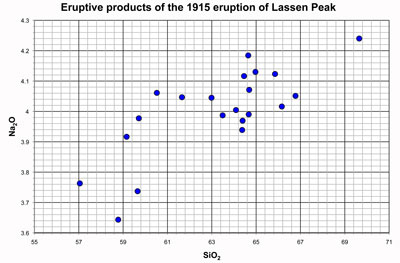Geochemical data from dacites erupted from Lassen Peak in 1915. Data collected by Rachelle Kernen, undergraduate student at University of Wisconsin Oshkosh and presented at the Fall Meeting of AGU in 2007.

2. Draw a shape that encloses all of the data, (try to make it smooth and relatively even).
3. Draw a line that divides the area that encloses the data in two even sized areas. In other words, bisect the area with a line that goes from one edge of the plot to the other.
4. Congratulations! You have just constructed a best fit line through the data!
Note that it is not necessary for the line to pass through ANY of the points on the plot, it is only important that your line bisect (cut in half) the area that encloses the data points. Now you can use the line to predict behavior. Or, you can examine the other method and try it out.
Note that the more tightly clustered the data are, the smaller the area is going to be. We can do the same thing with Al2O3 data from Lassen Peak and see the difference.
1. We begin by plotting Al2O3 data vs. SiO2. You can download and print this plot (Acrobat (PDF) 164kB Aug27 08) to use while you work through this exercise.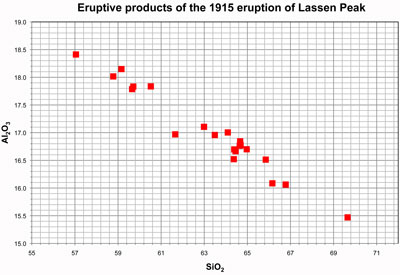Geochemical data from dacites erupted from Lassen Peak in 1915. Data collected by Rachelle Kernen, undergraduate student at University of Wisconsin Oshkosh and presented at the Fall Meeting of AGU in 2007.

2. Draw a shape that encloses all of the data.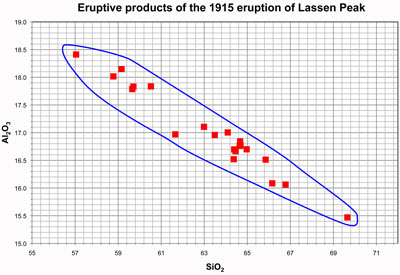Note that the area is smaller than in the Na plot above because there is less scatter in this data.
3. Draw a line that divides the area that encloses the data in two even sized areas. In other words, bisect the area with a line that goes from one edge of the plot to the other.
The second method involves dividing data into two equal groups, approximating the center of each group and constructing a line between the two centers.
1. Begin by plotting all of your data. For this exercise, we'll use the Na2O data from above.Geochemical data from dacites erupted from Lassen Peak in 1915. Data collected by Rachelle Kernen, undergraduate student at University of Wisconsin Oshkosh and presented at the Fall Meeting of AGU in 2007.

2. Draw a dotted line that divides the data in two (even numbers of points on either side of the line)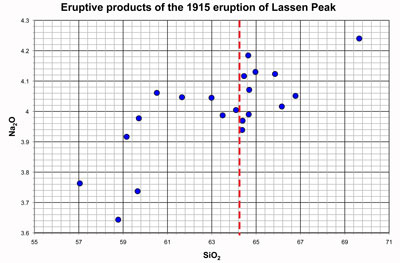In this case, there are 21 points on the graph, so, to the best of your ability, draw a line that has approximately 10.5 points on either side of it. There are three points that are really close to the line, so do your best.
3. Place an x (or a + or a dot) in your interpretation of the center of the data on either side of the line.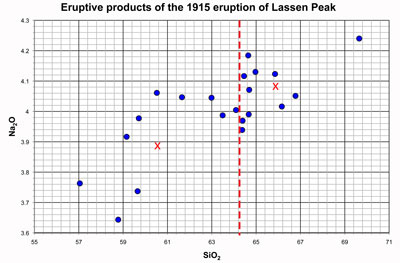Your x marks may not be in exactly the same place as mine - that's okay, we all see things slightly different. They shouldn't be too far off though.
4. Connect the x marks with a line that extends to the edges of the plot.
5. Congratulations! You have just constructed a best fit line through the data!
Note that it is not necessary for the line to pass through ANY of the points on the plot, it is only important that your x marks are in the center of the plotted data and your line connects those x marks. Now you can use the line to predict behavior. Or, you can examine the other method and try it out.
3. Evaluate your best fit line. Think back to the questions in number 1. Does your line look like you thought it should?
• Do you see that there are approximately the same number of data points on each side of the line?
• And are they evenly distributed (that is, make sure that plots with a variety of x values are on top (and bottom) of the line, not most above at the low end and most below at the high end)?
• Does your line minimize the average distance from it to each of the data points?
Note that in some cases, best-fit lines do not pass through anyof the points on the plots. It is not necessary to connect any dots when you are constructing a best-fit line.

You can also download and print a single sheet for constructing a best fit line with the area method (Acrobat (PDF) 33kB Sep10 08) or the dividing method (Acrobat (PDF) 34kB Sep10 08).

## Where is this used in the geosciences?

There are many instances in the geosciences where scientists use a best fit line. In the introductory geosciences, we use them for:
• flood frequency curves
• earthquake forecasting
• Meteorite impact prediction
• earthquake frequency vs. magnitude
• climate change

## Next steps

You have completed the construction of a best fit line! Now you can move on and practice with some other data on the sample problems page.